# How to Divide an Angle into Two Equal Angles

Instructor: Laura Pennington

Laura has taught collegiate mathematics and holds a master's degree in pure mathematics.

In this lesson, we will learn how to divide an angle into two equal parts. After seeing the steps to solve this type of problem, we will talk a bit about some real world situations in which this type of problem may arise.

## Steps to Solve

We want to know how to divide an angle into two equal parts. This is actually called bisecting an angle. When we bisect something, we divide it into two equal parts, and as we just said, that is exactly what we want to do to an angle. Luckily, to do this, it just takes a few materials and a few simple steps. The materials we need are a compass and a ruler. Simple enough, right?

Let's talk about the steps that we take to bisect an angle. We will illustrate these steps using the angle ABC in the following image.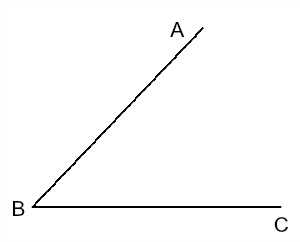The first thing we want to do is use our compass to draw an arc of any radius from point B that intersects the two sides of the angle. Label the intersection points D and E. This is shown in the following image.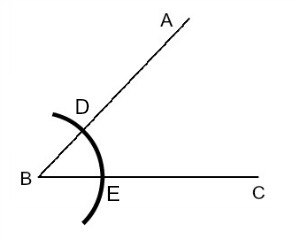Next, we want to draw two more arcs. One will start from point D and the other will start from point E. These two arcs should have equal radius and will intersect. Label this intersection point F. This is illustrated in the following image.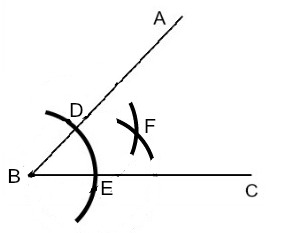Now, we have everything we need to divide our angle into two equal parts. The last step is to simply use a ruler to draw a line connecting points B and F. This line is called the bisector of angle ABC, and it divides angle ABC into two equal angles. In other words, angle ABF and angle FBC are equal. This is demonstrated in the following image.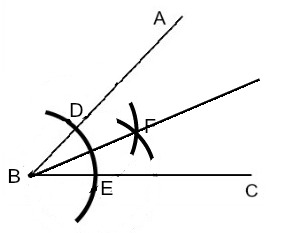That's not too hard, is it? Plus, it's pretty neat that we are able to accurately bisect an angle in just a few simple steps.

## Solution

To divide an angle ABC into two equal angles, we follow these steps.

1. Use a compass to draw an arc from B that intersects both sides of the angle. Label the intersection points D and E.
2. Use a compass to draw an arc from D and an arc from E. These arcs should have equal radius. Label the intersection point of these two arcs F.
3. Use a ruler to connect points B and F with a line. This line divides the angle into two equal angles, namely angle ABF and angle FBC.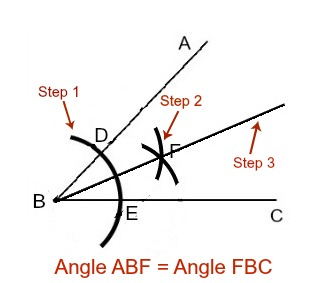## Real World Uses

Now that we know how to divide an angle into two equal parts, let's consider a couple of real world applications when this would be useful. The practice of bisecting an angle shows up often in quilting, sewing, architecture, carpentry, computer programming, and the like.

For example, have you ever taken the time to really look at the intricate design of a quilt? These beautiful blankets take on many different patterns and are quite visually stimulating.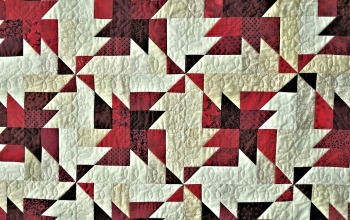To unlock this lesson you must be a Study.com Member.

### Register to view this lesson

Are you a student or a teacher?

#### See for yourself why 30 million people use Study.com

##### Become a Study.com member and start learning now.
Back
What teachers are saying about Study.com

### Earning College Credit

Did you know… We have over 200 college courses that prepare you to earn credit by exam that is accepted by over 1,500 colleges and universities. You can test out of the first two years of college and save thousands off your degree. Anyone can earn credit-by-exam regardless of age or education level.